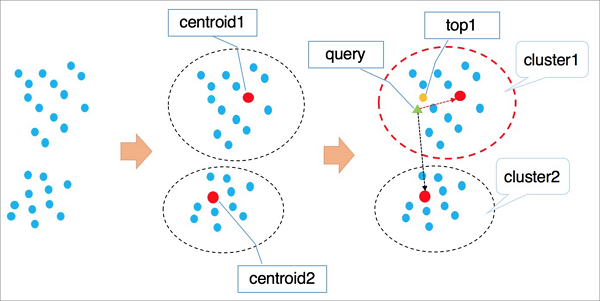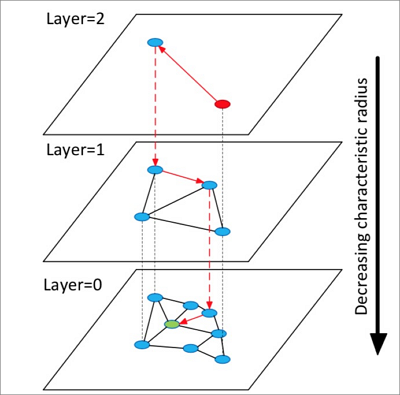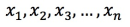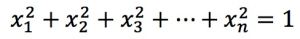This topic describes how to use the PostgreSQL ANN search extension (PASE) plug-in to search for vectors in RDS PostgreSQL.

## Background information

Representation learning is a typical artificial intelligence (AI) technology in the deep learning discipline. It has developed rapidly over the recent years and is used in various businesses such as advertising, face scan payment, image recognition, and voice recognition. This technology enables data to be embedded into high-dimensional vectors and allows you to query data by using the vector search approach.

PASE is a high-performance vector search index plug-in developed for PostgreSQL. It uses two well-developed, stable, and efficient approximate nearest neighbor (ANN) search algorithms, IVFFlat and Hierarchical Navigable Small World (HNSW), to query vectors from PostgreSQL databases at high speeds. PASE does not support the extraction or output of feature vectors. You must retrieve the feature vectors of the entities you want to query. PASE only implements a similarity search among a large amount of vectors identified based on retrieved feature vectors.

## Intended audience

This topic does not explain the terms related to machine learning in detail. Before you read this topic, you must understand the basics of machine learning and search technologies.

## Algorithms used by PASE

• IVFFlat
IVFFlat is a simplified version of the IVFADC algorithm. It is suitable for businesses that require high precision but can tolerate up to 100 milliseconds taken for queries. IVFFlat has the following advantages compared with other algorithms:
• If the vector to query is one of the candidate datasets, IVFFlat delivers 100% recall.
• IVFFlat uses a simple structure to create an index fast and occupy less storage space.
• You can specify a centroid for clustering and can control precision by reconfiguring parameters.
• You can control the accuracy of IVFFlat by reconfiguring its interpretable parameters.

The following figure shows how IVFFlat works.The procedure is described as follows:

1. IVFFlat uses a clustering algorithm such as k-means to divide vectors in the high-dimensional data space into clusters based on implicit clustering properties. Each cluster has a centroid.
2. IVFFlat traverses the centroids of all clusters to identify the n centroids nearest to the target vector you want to query.
3. IVFFlat traverses and sorts all vectors in the clusters to which the identified n centroids belong, and then obtains the nearest k vectors.
Note
• When IVFFlat attempts to identify the nearest n centroids, it skips the clusters located far away to expedite the query. However, IVFFlat cannot ensure that all the similar k vectors are included in the clusters to which the identified n centroids belong. As a result, precision may decrease. You can use the variable n to control precision. A larger n value indicates higher precision but more computing workloads.
• In the first phase, IVFFlat works the same as IVFADC. The main differences between them lie in the second phase. In the second phase, IVFADC uses product quantization to avoid traversal computing workloads. This is faster but has lower precision. Whereas, IVFFlat uses brute-force computing to ensure precision and allows you to control computing workloads.
• HNSW

HNSW is a graph-based ANN algorithm suitable for queries among tens of millions or more vector datasets that take 10 milliseconds or less.

HNSW searches among proximity graph neighbors for similar vectors. When the data volume is large, HNSW significantly improves performance compared to other algorithms. However, HNSW requires the storage of proximity graph neighbors, which occupy storage space. In addition, the precision of HNSW cannot be increased by reconfiguring parameters after reaching a certain level.

The following figure shows how HNSW works.The procedure is described as follows:

1. HNSW builds a hierarchical structure consisting of multiple layers (graphs). Each layer is a panorama and skip list of its lower layer.
2. HNSW randomly selects an element from the top layer to start a search.
3. HNSW identifies the neighbors of the selected element and adds the identified neighbors to a fixed-length dynamic list based on their distances to the selected element. HNSW continues to identify the neighbors of each neighbor included in the list and adds the identified neighbors to the list. Every time when HNSW adds a neighbor to the list, it re-sorts the neighbors in the list and only retains the first k neighbors. If the list changes, HNSW continues to search until the list reaches its final state. Then, HNSW uses the first element in the list as the start for a search in the lower layer.
4. HNSW repeats the third step until it enters the bottom layer.
Note HNSW constructs a multi-layer structure by using the Navigable Small World (NSW) algorithm designed to construct single-layer structures. The employment of an approach for selecting proximity graph neighbors enables HNSW to deliver higher query speedup than clustering algorithms.

IVFFlat and HNSW are each suitable for specific businesses. For example, IVFFlat is suitable for image comparison at high precision, and HNSW is suitable for searches with recommended recall. More industry-leading algorithms will be integrated into PASE.

## Procedure

1. Execute the following statement to create the PASE plug-in:
``CREATE EXTENSION pase;``
2. Use one of the following construction methods to calculate vector similarity:
• PASE-type-based construction

Example:

``````SELECT ARRAY[2, 1, 1]::float4[] <? > pase(ARRAY[3, 1, 1]::float4[]) AS distance;
SELECT ARRAY[2, 1, 1]::float4[] <? > pase(ARRAY[3, 1, 1]::float4[], 0) AS distance;
SELECT ARRAY[2, 1, 1]::float4[] <? > pase(ARRAY[3, 1, 1]::float4[], 0, 1) AS distance;``````
Note
• <? > is a PASE-type operator, which specifies to calculate similarity between the vectors to the left and right of a specific element. The vector to the left must use the float4[] data type and the vector to the right must use the PASE data type.
• The PASE data type is defined in the PASE plug-in and can contain up to three constructors. Take the `float4[], 0, 1` part in the preceding third statement as an example: The first parameter specifies the vector to the right with the float4[] data type. The second parameter does not serve a special purpose, so you can set it to 0. The third parameter specifies the similarity calculation method, where the value 0 represents the Euclidean distance method and the value 1 represents the dot product (also referred to as inner product) method.
• The vector to the left must have the same number of dimensions as the vector to the right. Otherwise, the system reports similarity calculation errors.
• String-based construction

Example:

``````SELECT ARRAY[2, 1, 1]::float4[] <? > '3,1,1'::pase AS distance;
SELECT ARRAY[2, 1, 1]::float4[] <? > '3,1,1:0'::pase AS distance;
SELECT ARRAY[2, 1, 1]::float4[] <? > '3,1,1:0:1'::pase AS distance;``````
Note The string-based construction method differs from the PASE-type-based construction in the following aspect: The string-based construction method uses colons (:) to separate parameters. Take the `3,1,1:0:1` part in the preceding third statement as an example: The first parameter specifies the vector to the right. The second parameter does not serve a special purpose, so you can set it to 0. The third parameter specifies the similarity calculation method, where the value 0 represents the Euclidean distance method and the value 1 represents the dot product (also referred to as the inner product) method.
3. Use IVFFlat or HNSW to create an index.
Note If you use the Euclidean distance method to calculate vector similarity, the original vector does not need to be processed. If you use the dot product or cosine method to calculate vector similarity, the original vector must be normalized. For example, if the original vector is, it must meet the following formula:. In this example, the dot product is the same as the cosine value.
• IVFFlat

Example:

``````CREATE INDEX ivfflat_idx ON vectors_table
USING
pase_ivfflat(vector)
WITH
(clustering_type = 1, distance_type = 0, dimension = 256, base64_encoded = 0, clustering_params = "10,100");``````

The following table describes the parameters in the IVFFlat index.

Parameter Description
clustering_type The type of clustering operation IVFFlat performs on vectors. This parameter is mandatory. Valid values:
• 0: external clustering. An external centroid file is loaded, which is specified by the clustering_params parameter.
• 1: internal clustering. The k-means clustering algorithm is used, which is specified by the clustering_params parameter.

If you are using PASE for the first time, we recommend that you use internal clustering.

distance_type The method to calculate vector similarity. Default value: 0. Valid values:
• 0: Euclidean distance.
• 1: dot product. This method requires the normalization of vectors. The order of dot products is opposite to the order of Euclidean distances.

Currently, only the Euclidean distance method is supported. Dot products can only be calculated after vectors are normalized. For more information, see Appendixes.

dimension The number of dimensions. This parameter is mandatory.
base64_encoded Specifies whether to use Base64 encoding. Default value: 0. Valid values:
• 0: specifies to use the float4[] data type to represent the vector type.
• 1: specifies to use the Base64-encoded float[] data type to represent the vector type.
clustering_params

For external clustering, this parameter specifies the directory of the external centroid file to use. For internal clustering, this parameter specifies the clustering algorithm to use. The format is as follows: `clustering_sample_ratio,k`. This parameter is mandatory.

• clustering_sample_ratio: the sampling fraction with 1000 as its denominator. The value of this field is an integer within the (0, 1000] range. For example, if you set this field to 1, the system samples data from the dynamic list based on the 1/1000 sampling ratio before it performs k-means clustering. A larger value indicates higher query accuracy but slower index creation. We recommend that the total number of data records sampled do not exceed 100,000.
• k: the number of centroids. A larger value indicates higher query accuracy but slower index creation. We recommend that you set this field to a value within the [100, 1000] range.
• HNSW

Example:

``````CREATE INDEX hnsw_idx ON vectors_table
USING
pase_hnsw(vector)
WITH
(dim = 256, base_nb_num = 16, ef_build = 40, ef_search = 200, base64_encoded = 0);``````

The following table describes the parameters in the HNSW index.

Parameter Description
dim The number of dimensions. This parameter is mandatory.
base_nb_num The number of neighbors to identify for an element. This parameter is mandatory. A larger value indicates higher query accuracy, but slower index creation and more storage space occupied. We recommend that you set this parameter to a value within the [16, 128] range.
ef_build The heap length to use during index creation. This parameter is mandatory. A longer heap length indicates higher query accuracy but slower index creation. We recommend that you set this parameter to a value within the [40, 400] range.
ef_search The heap length to use during query. This parameter is mandatory. A longer heap length indicates higher query accuracy but poorer query performance. You can specify this parameter when initiating the query request. Default value: 200.
base64_encoded Specifies whether to use Base64 encoding. Default value: 0. Valid values:
• 0: specifies to use the float4[] data type to represent the vector type.
• 1: specifies to use the Base64-encoded float[] data type to represent the vector type.
4. Use one of the following indexes to query a vector:
• IVFFlat index

Example:

``````SELECT id, vector <#> '1,1,1'::pase as distance
FROM vectors_ivfflat
ORDER BY
vector <#> '1,1,1:10:0'::pase
ASC LIMIT 10;``````
Note
• <#> is an operator used by the IVFFlat index.
• You must execute the ORDER BY statement to make the IVFFlat index take effect. The IVFFlat index allows vectors to be sorted in ascending order.
• The PASE data type requires three parameters to specify a vector. These parameters are separated with colons (:). For example, `1,1,1:10:0` includes three parameters: The first parameter specifies the vector to query. The second parameter specifies the query efficiency of IVFFlat with a value range of (0, 1000], in which a larger value indicates higher query accuracy but poorer query performance. The third parameter specifies the vector similarity calculation method, where the value 0 represents the Euclidean distance method and the value 1 represents the dot product (also referred to as inner product) method. The dot product method requires the normalization of vectors. The order of dot products is opposite to the order of Euclidean distances.
• HNSW

Example:

``````SELECT id, vector <? > '1,1,1'::pase as distance
FROM vectors_ivfflat
ORDER BY
vector <? > '1,1,1:100:0'::pase
ASC LIMIT 10;``````
Note
• <? > is an operator used by the HNSW index.
• You must execute the ORDER BY statement to make the HNSW index take effect. The HNSW index allows vectors to be sorted in ascending order.
• The PASE data type requires three parameters to specify a vector. These parameters are separated with colons (:). For example, `1,1,1:10:0` includes three parameters: The first parameter specifies the vector to query. The second parameter specifies the query efficiency of HNSW with a value range of (0, ∞), in which a larger value indicates higher query accuracy but poorer query performance. We recommend that you set the second parameter to 40 and then test the value by small increases until you find the most suitable value for your business. The third parameter specifies the vector similarity calculation method, where the value 0 represents the Euclidean distance method and the value 1 represents the dot product (also referred to as inner product) method. The dot product method requires the normalization of vectors. The order of dot products is opposite to the order of Euclidean distances.

## Appendixes

• Calculate the dot product of a vector.

For this example, use the HNSW index to create a function:

``````CREATE OR REPLACE FUNCTION inner_product_search(query_vector text, ef integer, k integer, table_name text) RETURNS TABLE (id integer, uid text, distance float4) AS \$\$
BEGIN
RETURN QUERY EXECUTE format('
select a.id, a.vector <? > pase(ARRAY[%s], %s, 1) AS distance from
(SELECT id, vector FROM %s ORDER BY vector <? > pase(ARRAY[%s], %s, 0) ASC LIMIT %s) a
ORDER BY distance DESC;', query_vector, ef,  table_name,  query_vector, ef, k);
END
\$\$
LANGUAGE plpgsql;``````
Note The dot product of a normalized vector is the same as its cosine value. Therefore, you can also follow this example to calculate the cosine value of a vector.
• Create the IVFFlat index from an external centroid file.

This is an advanced feature. You must upload an external centroid file to the specified directory of the server and use this file to create the IVFFlat index. For more information, see the parameters of the IVFFlat index. The file format is as follows:

``Number of dimensions|Number of centroids|Centroid vector dataset``

Example:

``3|2|1,1,1,2,2,2``